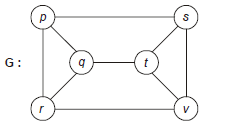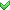##### What is the number of back edges during the above DFS traversal on G?

Consider the following graph G.Modified DFS on G applied as follows:
• Starting vertex is ‘p’.
• Vertex is visited based on alphabetic order.
• Vertices are visited in order p, q, r, s, t, v.
• It works same as DFS except the visiting order restriction

What is the number of back edges during the above DFS traversal on G?

Consider the following graph G.Modified DFS on G applied as follows:
• Starting vertex is ‘p’.
• Vertex is visited based on alphabetic order.
• Vertices are visited in order p, q, r, s, t, v.
• It works same as DFS except the visiting order restriction

What is the number of back edges during the above DFS traversal on G?

a. 2

b. 3

c. 4d. 5

Explain...